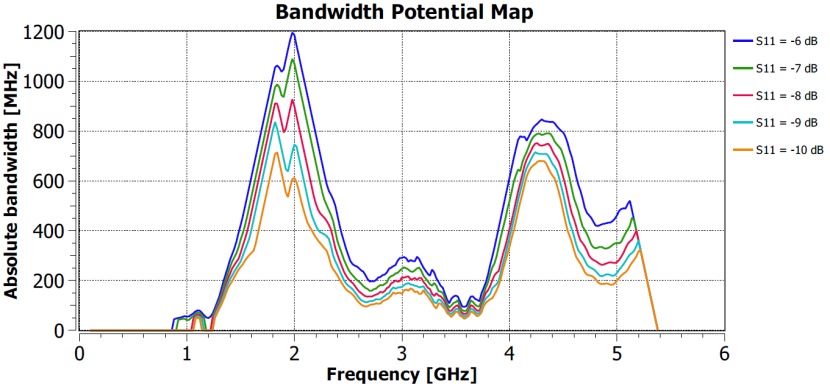# Optenni Lab: Estimation of obtainable bandwidth

#### Optenni Lab offers tools for estimating the obtainable bandwidth from antenna impedance curves using the bandwidth potential  and Q factor approaches.

###### Bandwidth potential

In the bandwidth potential calculation, Optenni Lab synthesizes for each frequency an optimal two-component matching circuit and calculates the obtained maximal impedance bandwidth. The analysis is carried out for all frequencies in the data, and results in a curve that shows the obtainable bandwidth at each center frequency.

With the bandwidth potential calculation you can

• Uncover ”hidden” bandwidth that may not be evident by looking at the impedance curve
• Verify if the bandwidth is large enough for the desired application even if the antenna was not originally resonant
• Make a proper bandwidth comparison of prototypes, factoring out easy-to-fix mismatch
• Check on which frequencies the antenna gives maximal bandwidth

The bandwidth potential calculation speeds up the antenna design process. You can quickly estimate the obtainable antenna bandwidth from a measured or simulated prototype without explicitly tuning the antenna to resonance at the desired frequency range. If the bandwidth is not sufficient, you can modify the design until the bandwidth potential meets the specifications, and only then consider the actuai implementation of the matching circuit.

Repeating the bandwidth potential analysis for different reference S11-levels, you can create a ”map” for a quick assessment of given antenna’s bandwidth behavior.Example of standard and optimized bandwidth potential. The vertical axis is relative bandwidth as a percentage of the center frequency.

###### Q factor estimation

In addition, Optenni Lab can estimate the antenna Q factor based on the impedance data directly. This method is less accurate than the bandwidth potential calculation especially if the impedance data is noisy or if multiple resonances are present.